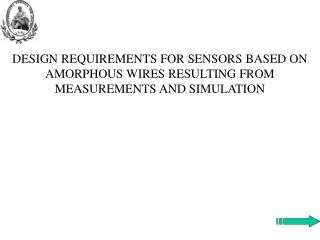Download PresentationIntroduction

# Introduction - PowerPoint PPT PresentationDownload Presentation## Introduction

- - - - - - - - - - - - - - - - - - - - - - - - - - - E N D - - - - - - - - - - - - - - - - - - - - - - - - - - -
##### Presentation Transcript

1. DESIGN REQUIREMENTS FOR SENSORS BASED ON AMORPHOUS WIRES RESULTING FROM MEASUREMENTS AND SIMULATION

2. Introduction • Amorphous wires are attractive components for sensor and micro-sensor realization. • The characteristics of the sensing element can be carried-out starting from the measurement results. • A model of a magneto-impedance sensing element was obtained and was used for a PSPICE simulation step. • The paper presents the results obtained at radio-frequency, at once with the mathematical modeling of the sensitive part. In addition, there are also the requirements which must be fulfilled in the design of this kind of sensors.

3. Current sensor based on magnetic amorphous wire

4. The fitting law from previous results

5. Ta ZLS HNF Hex I1 = 0.2 A I/H Z(Hex, IHF) I Z(Hex, IHF, Ta) HQ IHF Simulation Step Figure 4. Collpits oscillator for amorphous magnetic wire based on sensor Figure 3. Modeling of a current sensor with amorphous wire by two transducers; HQ - biasing magnetic field, HNF - negative feedback field

6. PSPICE simulation circuit Figure 5. Electrical diagram for PSPICE simulation

7. Simulation of GMI

8. 400mA 300mA 800mA I1 = 0.4 A 200mA 400mA I1 = 0.3 A I1 = 0.2 A I1 = 0.1 A I1 = 0 100mA 0A 0s 10us 20us Time I(F1) 0A 0 200mA I_I1 400mA (Min(I(F1))+Max(I(F1)))/2 (Min(I(H2))+Max(I(H2)))/2 Simulated Results Figure 6. PSPICE results of the feedback current for various input currents. Figure 7. Transfer characteristic of the feedback current IF1vs.I1.

9. 20W 31.8ns 15W 31.6ns 10W 31.4ns 5W 200mA 0 400mA I_I1 31.2ns 2*Max(V(E1:OUT+) - V(E1:OUT-))/(Max(I(H1)) - Min(I(H1))) 0 200mA 400mA I_I1 Period(I(H1)) Simulated Results Figure 8. Amorphous wire resistance variation vs.I1. Figure 9. Period of oscillation of the test current IH1vs.I1.

10. 9.0mA 10mA 8.0mA 100mA 7.0mA 6.0mA 0 200mA 400mA I_I1 Max(I(H1))/1.41 1.0mA 30MHz 50MHz 100MHz 130MHz Frequency I(H1) SimulatedResults Figure 10. Power spectral density of the test current IH1; parameter - I1. Figure 11. The effective value of the current IH1 through the amorphous wire vs.I1.

11. Conclusions • The characteristics of the amorphous wires can be theoretically modeled, but vectorial measurements are important for obtaining the fitting laws in the real conditions. • Starting from the experimental data , the mathematical, informational and energetic models are developed as a first step in PSPICE modeling for a current sensor based on magnetic amorphous wires. • In the simulation phase, magnetic amorphous wires based sensors nearly complete behaviors were obtained. • The resulted fast balancing loop, obtained by applying an incomplete compensation method, has good behaviors proved by many quality parameters. • Moreover, not easy to do in current measurements are obtained as function of the dc input quantity. • A compact non-contact current sensor and its electronic processing unit, designed on Collpits oscillator structure, can be developed starting from the presented simulation diagram. • The aim for the future is to extend the study of this current sensor in ac and to find the requirements for signal processing in this case.

12. References  E. Vremera, C. Fosalau, L. Brunetti, Instrumentation Set-Up for Characterization of the Sensors Based on Amorphous Wires, IMEKO TC4 Symposium, pp. 458-461, Gdinya (Poland), 2005  L. Brunetti, et al., “Magneto-impedance measurements in amorphous Co-based magnetic wires at high frequency”, Journal of Magnetism and Magnetic Materials, no. 249, pp. 310–314, 2002.  K. Bushida, et al., Sensitive and Quick Response Micro Magnetic Sensor Using Amorphous Wire MI Element Collpits Oscillator, IEEE Transaction on Magnetics, vol. 31, no. 6, pp. 3134-3136, 1995  A.F.P. van Putten, Electronic Measurement Systems, Institute of Physics Publishing, 1996.  F. Monssen, MicroSim PSpice with circuit analysis - 2nd ed. - Prentice Hall, 1998.